## 3.10 Making a Cleveland Dot Plot

### 3.10.1 Problem

You want to make a Cleveland dot plot.

### 3.10.2 Solution

Cleveland dot plots are an alternative to bar graphs that reduce visual clutter and can be easier to read.

The simplest way to create a dot plot (as shown in Figure 3.28) is to use `geom_point()`:

``````library(gcookbook) # Load gcookbook for the tophitters2001 data set
tophit <- tophitters2001[1:25, ] # Take the top 25 from the tophitters data set

ggplot(tophit, aes(x = avg, y = name)) +
geom_point()``````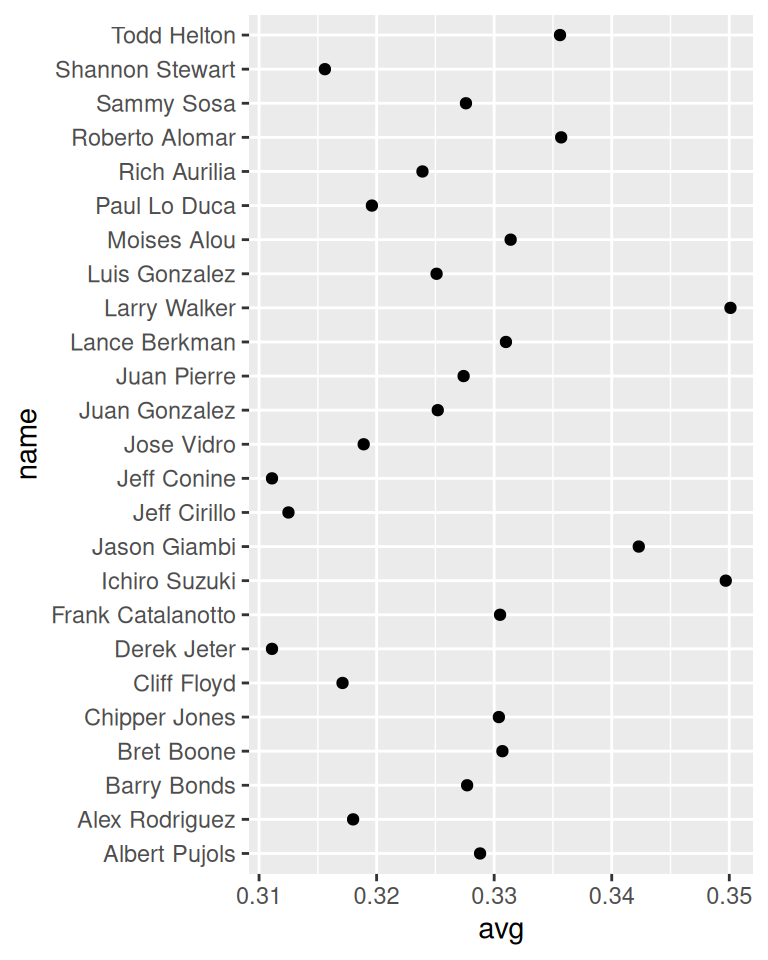Figure 3.28: Basic dot plot

### 3.10.3 Discussion

The `tophitters2001` data set contains many columns, but we’ll focus on just three of them for this example:

``````tophit[, c("name", "lg", "avg")]
#>             name lg    avg
#> 1   Larry Walker NL 0.3501
#> 2  Ichiro Suzuki AL 0.3497
#> 3   Jason Giambi AL 0.3423
#>  ...<19 more rows>...
#> 23  Jeff Cirillo NL 0.3125
#> 24   Jeff Conine AL 0.3111
#> 25   Derek Jeter AL 0.3111``````

In Figure 3.28 the names are sorted alphabetically, which isn’t very useful in this graph. Dot plots are often sorted by the value of the continuous variable on the horizontal axis.

Although the rows of `tophit` happen to be sorted by `avg`, that doesn’t mean that the items will be ordered that way in the graph. By default, the items on the given axis will be ordered however is appropriate for the data type. `name` is a character vector, so it’s ordered alphabetically. If it were a factor, it would use the order defined in the factor levels. In this case, we want `name` to be sorted by a different variable, `avg`.

To do this, we can use `reorder(name, avg)`, which takes the name column, turns it into a factor, and sorts the factor levels by `avg`. To further improve the appearance, we’ll make the vertical grid lines go away by using the theming system, and turn the horizontal grid lines into dashed lines (Figure 3.29):

``````ggplot(tophit, aes(x = avg, y = reorder(name, avg))) +
geom_point(size = 3) +  # Use a larger dot
theme_bw() +
theme(
panel.grid.major.x = element_blank(),
panel.grid.minor.x = element_blank(),
panel.grid.major.y = element_line(colour = "grey60", linetype = "dashed")
)``````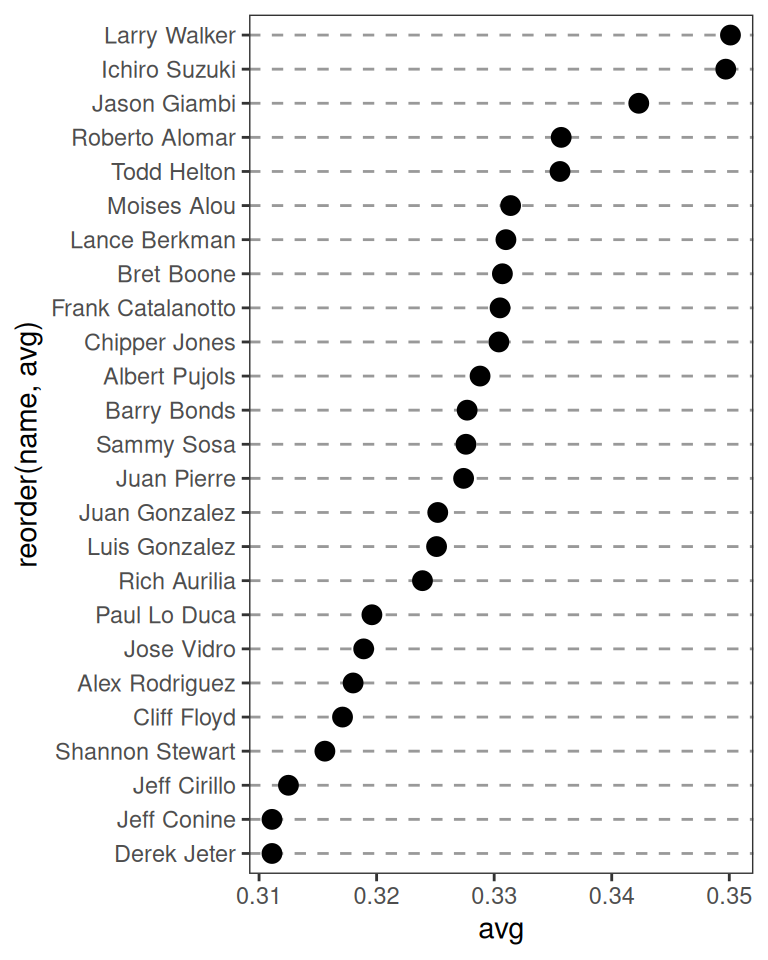Figure 3.29: Dot plot, ordered by batting average

It’s also possible to swap the axes so that the names go along the x-axis and the values go along the y-axis, as shown in Figure 3.30. We’ll also rotate the text labels by 60 degrees:

``````ggplot(tophit, aes(x = reorder(name, avg), y = avg)) +
geom_point(size = 3) +  # Use a larger dot
theme_bw() +
theme(
panel.grid.major.y = element_blank(),
panel.grid.minor.y = element_blank(),
panel.grid.major.x = element_line(colour = "grey60", linetype = "dashed"),
axis.text.x = element_text(angle = 60, hjust = 1)
)``````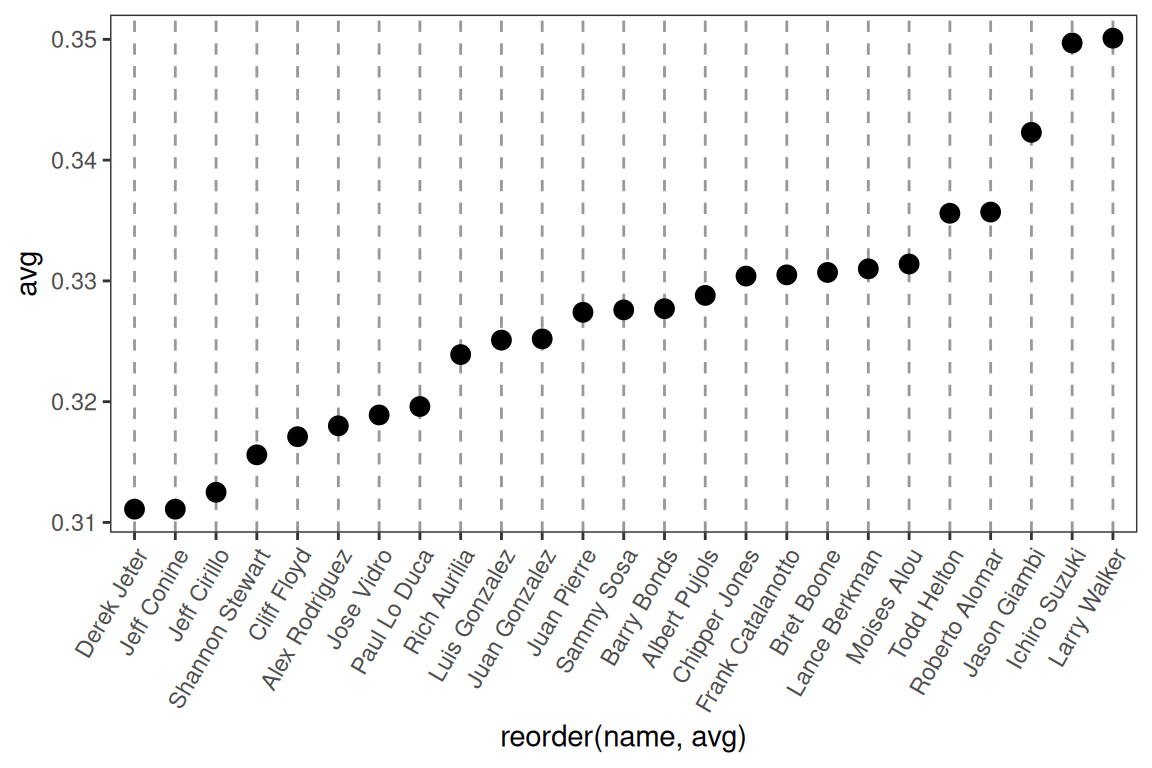Figure 3.30: Dot plot with names on x-axis and values on y-axis

It’s also sometimes desirable to group the items by another variable. In this case we’ll use the factor `lg`, which has the levels `NL` and `AL`, representing the National League and the American League. This time we want to sort first by `lg` and then by `avg`. Unfortunately, the `reorder()` function will only order factor levels by one other variable; to order the factor levels by two variables, we must do it manually:

``````# Get the names, sorted first by lg, then by avg
nameorder <- tophit\$name[order(tophit\$lg, tophit\$avg)]

# Turn name into a factor, with levels in the order of nameorder
tophit\$name <- factor(tophit\$name, levels = nameorder)``````

To make the graph (Figure 3.31), we’ll also add a mapping of `lg` to the color of the points. Instead of using grid lines that run all the way across, this time we’ll make the lines go only up to the points, by using `geom_segment()`. Note that `geom_segment()` needs values for `x`, `y`, `xend`, and `yend`:

``````ggplot(tophit, aes(x = avg, y = name)) +
geom_segment(aes(yend = name), xend = 0, colour = "grey50") +
geom_point(size = 3, aes(colour = lg)) +
scale_colour_brewer(palette = "Set1", limits = c("NL", "AL")) +
theme_bw() +
theme(
panel.grid.major.y = element_blank(),   # No horizontal grid lines
legend.position = c(1, 0.55),           # Put legend inside plot area
legend.justification = c(1, 0.5)
)``````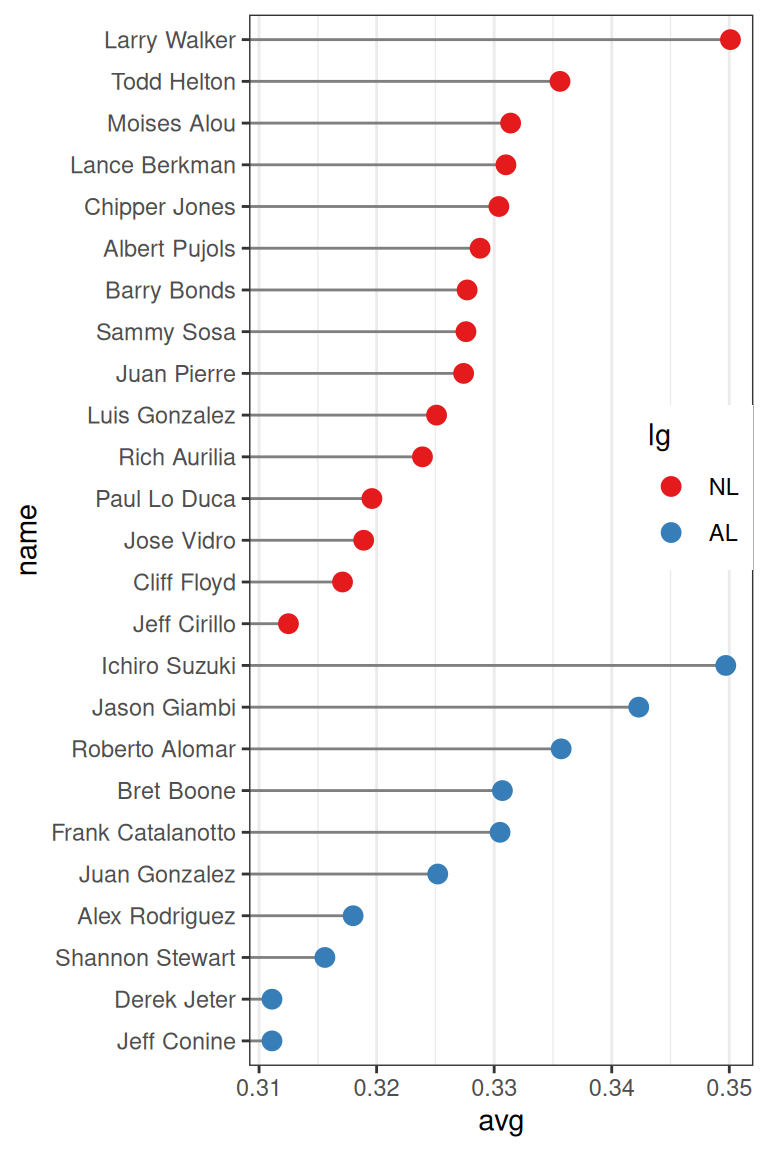Figure 3.31: Grouped by league, with lines that stop at the point

Another way to separate the two groups is to use facets, as shown in Figure 3.32. The order in which the facets are displayed is different from the sorting order in Figure 3.31; to change the display order, you must change the order of factor levels in the `lg` variable:

``````ggplot(tophit, aes(x = avg, y = name)) +
geom_segment(aes(yend = name), xend = 0, colour = "grey50") +
geom_point(size = 3, aes(colour = lg)) +
scale_colour_brewer(palette = "Set1", limits = c("NL", "AL"), guide = FALSE) +
theme_bw() +
theme(panel.grid.major.y = element_blank()) +
facet_grid(lg ~ ., scales = "free_y", space = "free_y")``````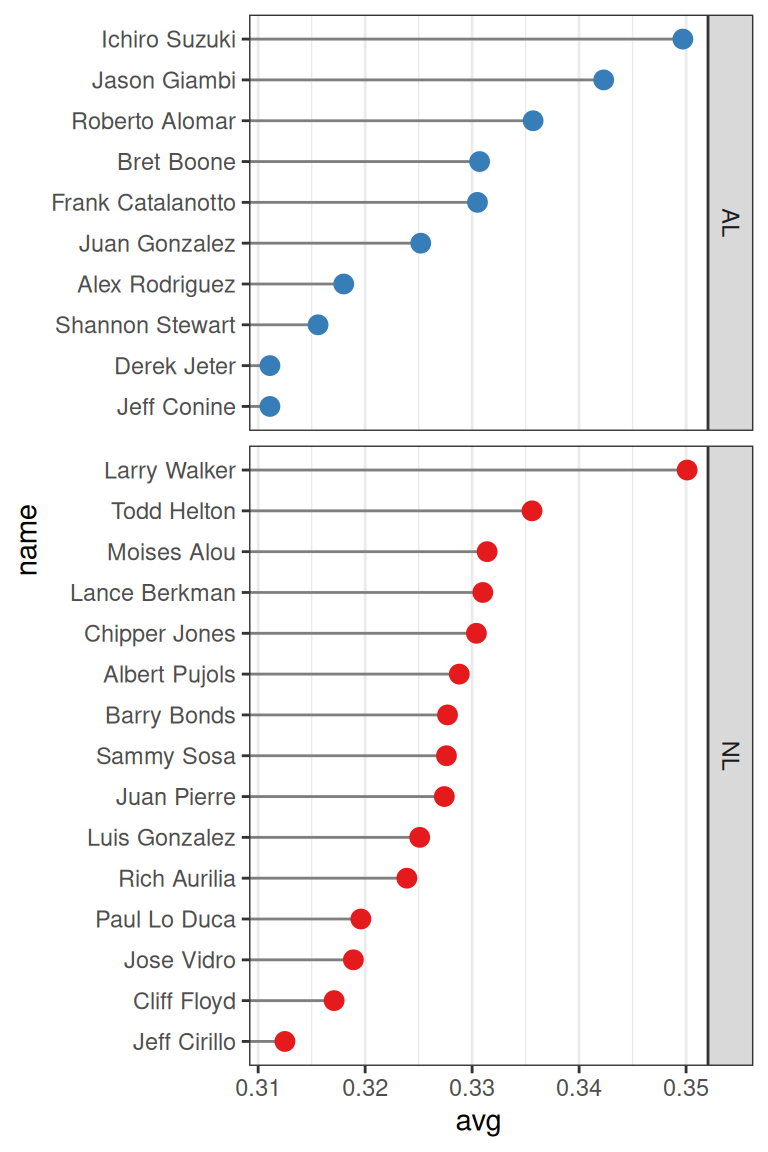Figure 3.32: Faceted by league